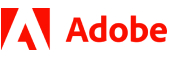New update is available. Click here to update.
Topics

# Successor Problem

Moderate0/80
Average time to solve is 32m## Problem statement

Coding Ninjas has given you an integer 'N'. You have to print the number succeeded by the given number in the lexicographically sorted permutation of all digits of the given number.

If the number is the last element of lexicographically sorted permutation of all digits of the given number, then print -1.

For Example:

``````The lexicographically sorted list of permutations of all digits of the number ‘123’ is:
123
132
213
231
312
321.

If we are given N as 123 then the permutation which precedes 123 is 132.

Similarly, if N is 132 then the permutation which precedes 132 is 213.

For 321 there does not exist any permutation greater than 321. So we need to print -1.
``````
Detailed explanation ( Input/output format, Notes, Images )
Constraints:
``````1 <= T <= 10 ^ 5
0 <= N <= 10 ^ 17

Time Limit: 1 sec.
``````
Sample Input 1:
``````1
112
``````
Sample Output 1:
``````121
``````
Explanation of the Sample Input 1:
``````From  112 we can have permutations 112, 121, and 211.
The number which precedes 112 is 121.
``````
Sample Input 2 :
``````2
402356
321
``````
Sample Output 2 :
``````402365
-1
``````Console